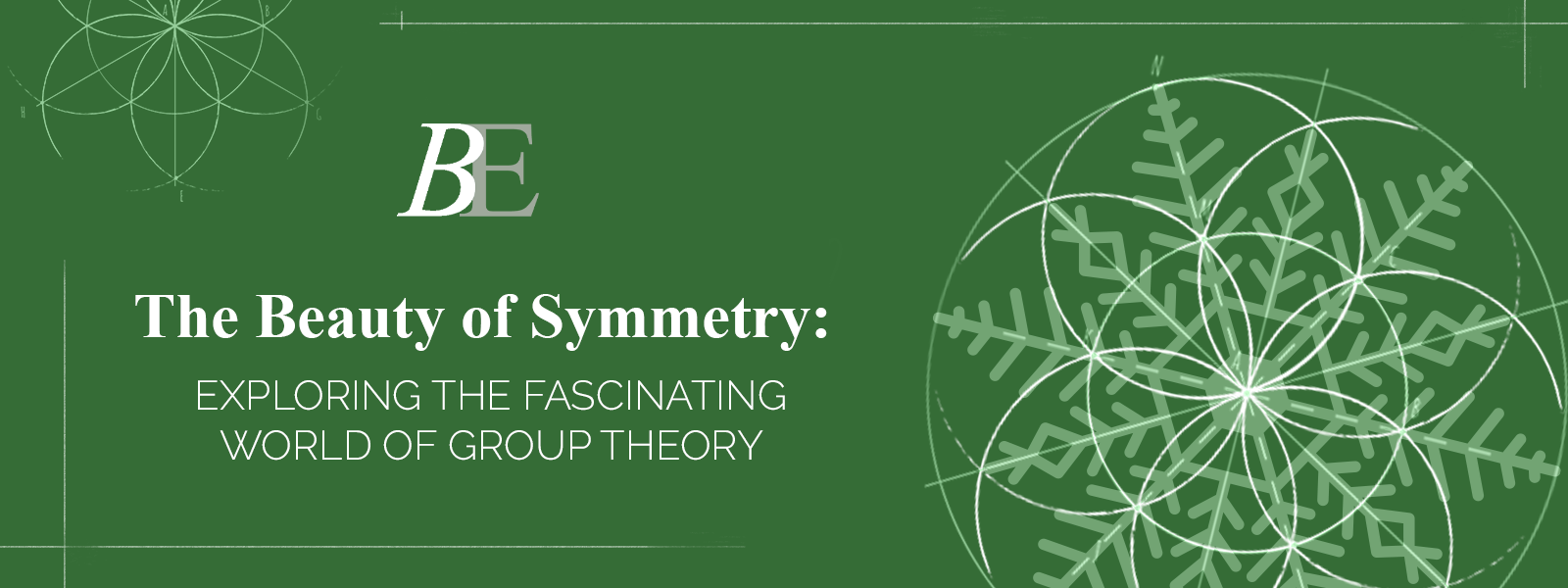## The Beauty of Symmetry: Exploring the Fascinating World of Group Theory

If you have ever looked at a snowflake or a kaleidoscope and felt a sense of wonder at their perfect symmetrical patterns, you have already appreciated the beauty of symmetry. But did you know that symmetry is not just a pretty face, but a fundamental concept in mathematics that has far-reaching implications in various fields, from physics to computer science?

Enter group theory, the branch of mathematics that studies symmetry and the structures that preserve it. In this post, we will take a peek into the fascinating world of group theory and discover some of its most interesting applications and insights.

First, let's define what we mean by a group. In mathematics, a group is a set of elements that can be combined or "operated" in a certain way, such that the combination satisfies certain properties, namely:

1. Closure: the result of combining any two elements in the group is also an element of the group.
2. Associativity: the way we combine three or more elements does not depend on the order in which we combine them.
3. Identity: there exists an element in the group that, when combined with any other element, leaves that element unchanged.
4. Inverse: for every element in the group, there exists another element in the group that, when combined with the first, gives the identity element.

These properties may seem abstract, but they capture the essence of what it means to have a symmetry-preserving structure. For example, consider the group of rotations of a regular polygon. The elements of the group are the rotations by certain angles (such as 90 degrees or 120 degrees), and the operation is composition: if we rotate by one angle and then by another, we get a third rotation that corresponds to the composition of the first two. This group satisfies the four properties above, and it is a classic example of a finite group (one that has a finite number of elements).

But group theory goes far beyond finite groups and polygons. In fact, group theory has found applications in fields as diverse as cryptography, quantum mechanics, and music theory. For example, the RSA algorithm, which is widely used for secure online communication, relies on the fact that certain mathematical operations can be performed efficiently in a group, but are hard to reverse without knowing some secret information (such as a prime factorization).

In physics, group theory plays a central role in understanding the symmetries of the universe. The famous physicist Emmy Noether showed that every symmetry of a physical system corresponds to a conserved quantity (such as energy or momentum), and vice versa. This deep connection between symmetry and conservation laws has led to many breakthroughs in modern physics, from the theory of relativity to the standard model of particle physics.

In music theory, group theory can help us understand the relationships between different chords and scales. For example, the major scale and the minor scale are related by a transformation called a "mode change," which corresponds to a certain element of a group of symmetries of the octave. By studying these symmetries, we can gain a deeper appreciation for the beauty and complexity of music.

In conclusion, group theory is a fascinating and powerful tool for understanding symmetry and its applications in many areas of mathematics and beyond. Whether you are a mathematician, a physicist, a musician, or simply a lover of patterns and symmetry, group theory offers a rich and rewarding world to explore.